Name:    Chapter 1 Post Test

Multiple Choice
Identify the choice that best completes the statement or answers the question.

1.

Plot the number 2.8 on the real number line.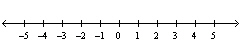a.b.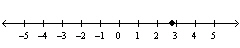c.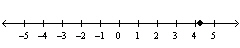d.e.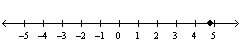2.

Which of the following is an example of a rational number that is not a natural number?
 a. –9 b.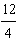c.d. 9 e.3.

Evaluate the expression.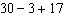a. 44 b. 16 c. 50 d. 10 e. –10

4.

Find the difference.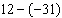a. –19 b. –9 c. 43 d. –43 e. 9

5.

What number must be added to 38 to obtain –7?
 a. 31 b. –31 c. –45 d. 45 e. 76

6.

The temperature at 6 A.M. was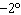F. By noon, the temperature had increased by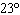F. What was the temperature at noon?
 a. 23°F b. 21°F c. 25°F d. 2°F e. –25°F

7.

A man awoke to a temperature of 40°F. By the time he arrived at work, the temperature had increased by 11°F. At lunch he noted the temperature had decreased from the temperature when he arrived at work by 12°F. Upon arriving home after work, the temperature had decreased another 13°F. What was the temperature when the man arrived home?
 a. 76°F b. 26°F c. 52°F d. 15°F e. 4°F

8.

The temperature measured by a weather balloon is decreasing approximately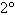F for each 1000-foot increase in altitude. The balloon rises 9000 feet. What is the total temperature change?
 a.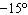F b.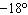F c.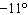F d.F e.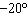F

9.

An object moves a distance of 490 miles in 7 hours. What is the object's average speed in miles per hour?
 a.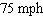b.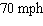c.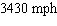d.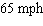e.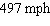10.

Add the fractions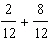and write the result in simplest form.
 a.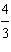b.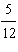c.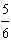d.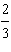e.11.

Multiply the fractionsand write the result in simplest form.
 a.b.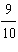c.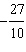d.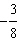e.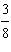12.

Multiply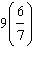and write the result in simplest form.
 a.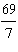b.c.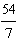d.e.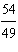13.

Write the fraction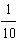in decimal form.
 a. 0.6 b. 0.2 c. 9.9 d. 9.4 e. 0.1

14.

Rewrite the expression in exponential form.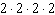a.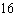b.c.d.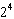e.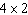15.

Evaluate the expression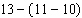.
 a.b.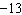c.d.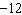e.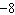16.

Evaluate the expression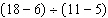, if possible.
 a.b.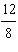c.d.e. not possible

17.

Evaluate the expression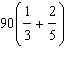.
 a.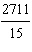b.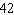c.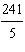d.e.18.

Identify the property of real numbers illustrated by the statement.
 a. Commutative Property of Multiplication b. Commutative Property of Addition c. Distributive Property d. Associative Property of Addition e. Associative Property of Multiplication

19.

Rewrite the expression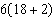by using the Distributive Property of real numbers.
 a.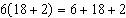b.c.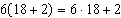d.e.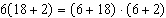20.

Find the additive inverse and the multiplicative inverse of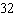.
 a. additive inverse: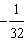multiplicative inverse:b. additive inverse:multiplicative inverse: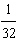c. additive inverse: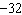multiplicative inverse:d. additive inverse:multiplicative inverse:e. additive inverse:multiplicative inverse: